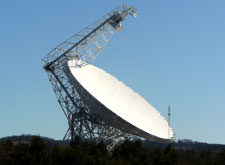# GBT 4mm Receiver Call for Proposals

The GBT 4mm system is a traditional dual-beam feed horn receiver designed to cover the low-frequency end of the 3mm atmospheric window from 67-93 GHz (GBT 4mm Receiver).

### System Performance

• The frequency range of the system is currently 67--93 GHz.
• The receiver temperatures are 30-100 K from 67-93 GHz. See the Figure below for the performance as a function of frequency for performance in 2013. For the 2015 fall season, we plan to adjust the optimization of the LNA's of the 4mm reciever to enable more efficient observations of the N2H+ line at 93.17 GHz. We should have better Trx performance above 90 GHz at the expense of small degredation of receiver performance below 70 GHz (which is dominated by ATM O2 anyway).
• The IF system for the 4mm system is broken into four separate filter bands:
• FL1: 67-74 GHz
• FL2: 73-80 GHz
• FL3: 79-86 GHz
• FL4: 85-93 GHz,
You can only use one of these bands at a time (i.e., you cannot simultaneously observe lines in more than one band).
• The millimeter down-converter filters of the system limits the instantaneous bandwidth to 4 GHz for 73-93 GHz (filters FL2, FL3,FL4), while 6 GHz of bandwidth is available for 67-74 GHz (filter FL1). ( IF-system ).
• The FWHM beam size is about 9 arcsec at 90 GHz.
• The separation of the two beams is 286 arcsec (4.77') along the cross-elevation (azimuth) direction.
• The aperture efficiency for night-time observations is expected to be about 32% at 90 GHz. The surface performance is better at lower frequencies.
• In general, continuum observations should be done with Mustang.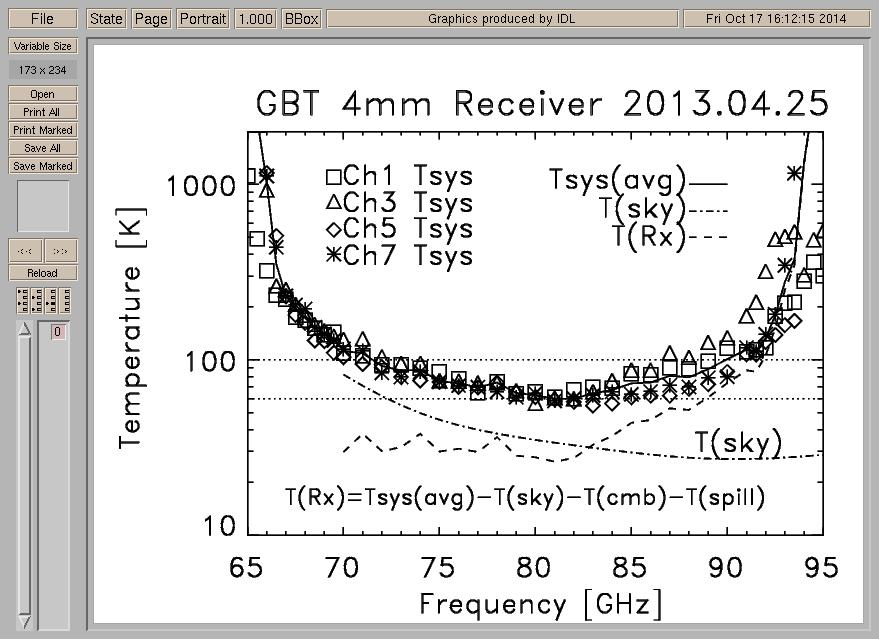Above shows the measured system temperatures for the 4 channels (2 beams and 2 polarizations) as symbols. The solid line is the average(median) Tsys for 4 channels as a function of frequency. The dashed-dotted line shows the contribution of the atmosphere at the time of the obseravations. The dashed line is the inferred receiver temperature (T(rx) = Tsys - T(sky) - T(cmb) - T(spill), where T(cmb) is the 2.7 K cosmic background and T(spill)=2.8 K is the estimated contribution to the system temperature from spill-over. The dotted-lines (100K and 60K) are reference lines to show the performance over the middle of the band. The Tsys goes up at low frequency due to the Atmospheric O_2 line, while the high-end of the band is limited by the receiver. Beam-2 (ch5 and ch7) has better performance within the 85-90 GHz frequency range.

### Sensitivity Estimate

The current performance levels have been incorporated into the GBT sensitivity calculator and should be used to estimate observing times. (Sensivity calculator)

For a sanity check of the approximate sensitivity expected, the noise on the T_A temperature scale for ON-OFF observations is
sigma(T_A) = 2*Tsys(np*dnu*t)^(-0.5),
where Tsys is the system temperature in K, np is the number of polarizations, dnu is the bandwidth in Hz, and t is the time in seconds for the ON+OFF observations. For 1hr of ON+OFF observations, dnu=1MHz, np=2, and Tsys=125K, sigma(T_A) =~ 3mK.

Correcting for the atmosphere, the noise on the main beam temperature scale T_mb for sources larger than the beam is
sigma(T_mb) = sigma(T_A)*exp(tau_o*Airmass)/eta_mb,
where tau_o is the zenith opacity and eta_mb is the main beam efficiency which is about 1.32 times that of the aperture efficiency for the GBT (but eta_mb still needs to be measured and verified at 4mm). For the same sigma(T_A) =3mK (1hr of ON+OFF, dnu=1MHz, np=2, and Tsys=125K), tau_o=0.1, Airmass=1.4, and eta_mb = 0.42 for an aperture efficiency of 32% at 90 GHz, sigma(T_mb) =~ 8 mK.

For point sources, the noise in flux density units is
sigma(S_nu) = 2k*sigma(T_A)*exp(tau_o*Airmass)/(eta_a*A_GBT),
where k is the Boltzmann constant, eta_a is the aperture efficiency, and A_GBT = the projected area of the telescope (7854.0 m^2). For the same sigma(T_A) =3mK (1hr of ON+OFF, dnu=1MHz, np=2, and Tsys=125K), tau_o=0.1, Airmass=1.4, and eta_a = 0.32 (at 90GHz), sigma(S_nu) =~ 4 mJy.

For estimating the total amount of time required, include about 10 minutes for session setup, add 10 minutes per hour for the standard point+focus and the hot+cold load calibration sequence, 10 minutes per session for absolute flux calibration, and 30 minutes per 2-4 hours for the AutoOOF procedure.

The ongoing status of the instrument will be reported on the (GBT 4mm Receiver Web Page). Feel free to contact David Frayer if you have any questions: ().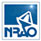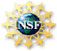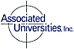The National Radio Astronomy Observatory is a facility of the National Science Foundation operated under cooperative agreement by Associated Universities, Inc.

Last updated 17 July 2015 by David Frayer